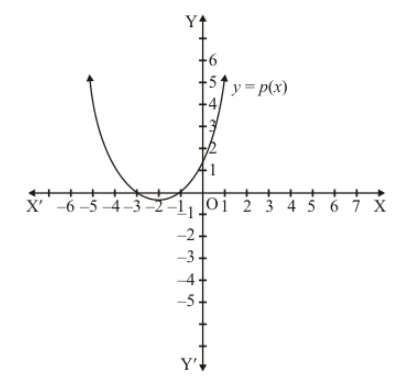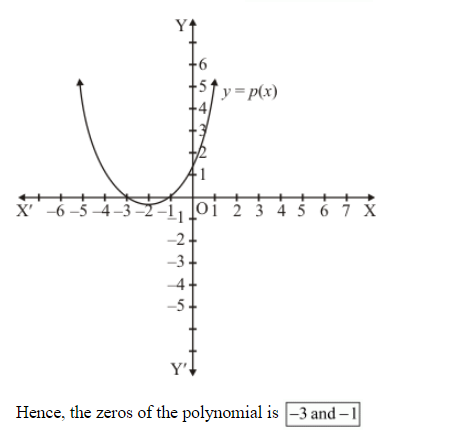# In Fig. 2.17, the graph of a polynomial p(x) is given. Find the zeros of the polynomial.

Question:

In Fig. 2.17, the graph of a polynomial p(x) is given. Find the zeros of the polynomial.Solution:

Just see the point of intersection of the curve and x-axis and find out the x-coordinate of these points. These x-coordinates will be the zeros of the polynomial

Since the intersection points are  $(-3,0)$ and $(-1,0)$